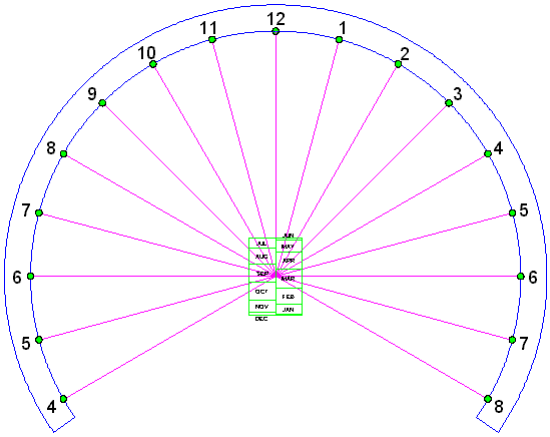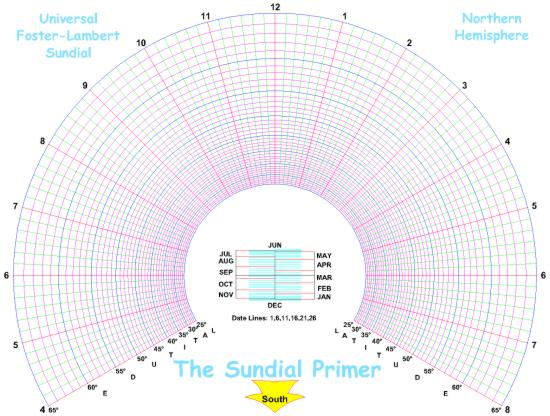The Sundial Primer created by Carl SabanskiThe Sundial Primer Index
 Universal Foster-Lambert Sundial Foster-Lambert Sundial: a form of equatorial projection dial, with the projection arranged to produce a circular ring of equiangular hour points. Figure 1 illustrates a typical Foster-Lambert sundial with its scale of dates. The spacing of the hour points is equiangular and located on the circumference of a circle. The gnomon for this sundial is sloped dependent upon the latitude of the place where the sundial is to be located.Figure 1: Foster-Lambert Sundial (CAD) The position of the point of any given date on the scale of dates can be determined as follows: Y = E * tan (dec)where "dec" is the sun's declination.The value of "E" is determined as follow:E = R / tan A = R * tan (90 - A) where "R" is the radius of the circle where the hour points are located and "A" is the angle the gnomon makes with the horizontal dial plate. "A" is determined as follows:A = (90 + ø) / 2 where ø is the latitude. From the above it can be seen that the scale of dates is latitude dependent.For more details check out the "Foster-Lambert Sundial" page.It is possible to create a universal Foster-Lambert sundial. To do this the equation for the scale of dates must be made independent of the latitude "ø".  This is done by making "E" a constant. The scale of dates is now dependent upon only the sun's declination for any latitude.However, for "E" to be a constant the radius "R" for the hour point circle will change with latitude as follows:R = E * tan AFigure 2 illustrates the layout of a universal Foster-Lambert sundial. Although it may appear there are hour lines, the time is determined by the point the gnomon's shadow falls on for a particular latitude circle. This sundial will operate in the latitude range of 25° to 65° in the Northern Hemisphere. For the Southern Hemisphere the hour numbers will be reversed and the scale of dates will be a mirror image about the horizontal axis through the centre of the dial. The "South" arrow will now be "North".Figure 2: Universal Foster-Lambert Sundial Layout (CAD) The angle of the gnomon will also have to be adjustable through the range of 57.5º to 77.5º for this latitude range.